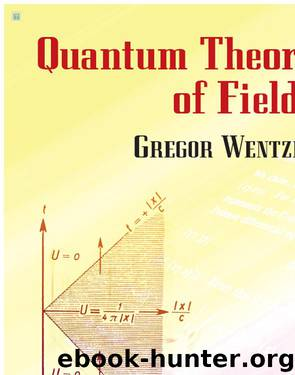# QUANTUM THEORY OF FIELDS by UnknownAuthor:Unknown
Language: eng
Format: epub, azw3
Published: 2019-11-20T16:00:00+00:00

1 First investigated (without quantization) by Proca, J. phys. radium 7, 347, 1936.

2 With the Lagrangian:

which yields (12.1) without (12.2), one obtains as Hamiltonian:

the term with ν = 4 in the sum is negative, since ψ4 = iψ0 (ψ0 real).

3 Such charged particles of negative energy would carry out transitions into states of higher momentum under emission of light. We shall discuss later why negative energy states are permissible for the Dirac electron, but not in our case (§§20 to 22).

4 L is independent of and . We have drawn attention to this case in §1.

5 Since the identically vanishing function π4 does not appear in H, H does not yield the correct canonical field equation for ; but one can succeed by adding formally to H certain terms which contain π4. We shall not discuss this, since the following elimination of ψ4, will lead to the same result.

6 a · b signifies the scalar, a × b the vector product of the 3-component vectors a and b.

7 The generalization of this formula for the case in question (several complex field components) is trivial. About the momentum density see below.

8 In the unquantized theory, a+ and a− represent the amplitudes of circular vibrations.

9 See footnote 1, p. 75.

10 For the current density: